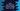# Java program to find Harshad or Niven number from 1 to 100This tutorial is to find Harshad or Niven number in Java. A Harshad or number or even number is a number that is divisible by its digits.

For example, 63 : The sum of its digits is_ 6+3 = 9. 63_ is divisible by 9 . So it is a Harshad number. But 64 is not. Because 6+4 =10 and_ _64%10__ or reminder is not zero. We will write one Java program to find all Harshad numbers starting from 1 to 100.

All one digits numbers are Harshad number, because each number is divided by that number.

### Java program to find all Harshad/Niven numbers from 1 to 100 :

``````/*
*
* you may not use this file except in compliance with the License.
* You may obtain a copy of the License at
*
*
* Unless required by applicable law or agreed to in writing, software
* WITHOUT WARRANTIES OR CONDITIONS OF ANY KIND, either express or implied.
* See the License for the specific language governing permissions and
*/

/**
* Class to find Harshad / Niven number from 1 to 100
*/
public class Test {

static void print(String value) {
System.out.print(value);
}

/**
* find sum of digits of a number
*
* @param number : number to find the sum of its digit
* @return : sum of all digits
*/
static int findSumOfDigits(int number) {
int sum = 0;
while (number > 0) {
sum += number % 10;
number /= 10;
}
return sum;
}

public static void main(String[] args) {

for (int i = 1; i < 101; i++) {
if (i % findSumOfDigits(i) == 0) { //if sum of digits can divide the number, then it is a Harshad number
print(i + " ");
}
}

}
}``````

### Output :

``1 2 3 4 5 6 7 8 9 10 12 18 20 21 24 27 30 36 40 42 45 48 50 54 60 63 70 72 80 81 84 90 100 ``